# RD Sharma Solutions for Class 9 Maths Chapter 19 Surface Area and Volume of A Right Circular Cylinder

## RD Sharma Solutions Class 9 Maths Chapter 19 – Free PDF Download

RD Sharma Solutions for Class 9 Maths Chapter 19 Surface Area and Volume of A Right Circular Cylinder provides in-depth knowledge of each concept in accordance with student’s intelligence quotient. In this chapter, students will learn how to find the surface area and volume of a cylinder. RD Sharma Solutions for Class 9 Chapter 19 are given here so that students grasp the important concepts with ease and prepare for exams more effectively.

Class 9 is a stage where several important topics are introduced. These crucial topics are discussed here and are formulated by BYJU’S experts in Maths. The RD Sharma Solutions are provided in accordance with the latest syllabus of CBSE updated for 2021-22 which, in turn, helps students to build a strong foundation of basics and secure excellent marks in their board exams.

## Download PDF of RD Sharma Solutions for Class 9 Maths Chapter 19 Surface Area and Volume of A Right Circular Cylinder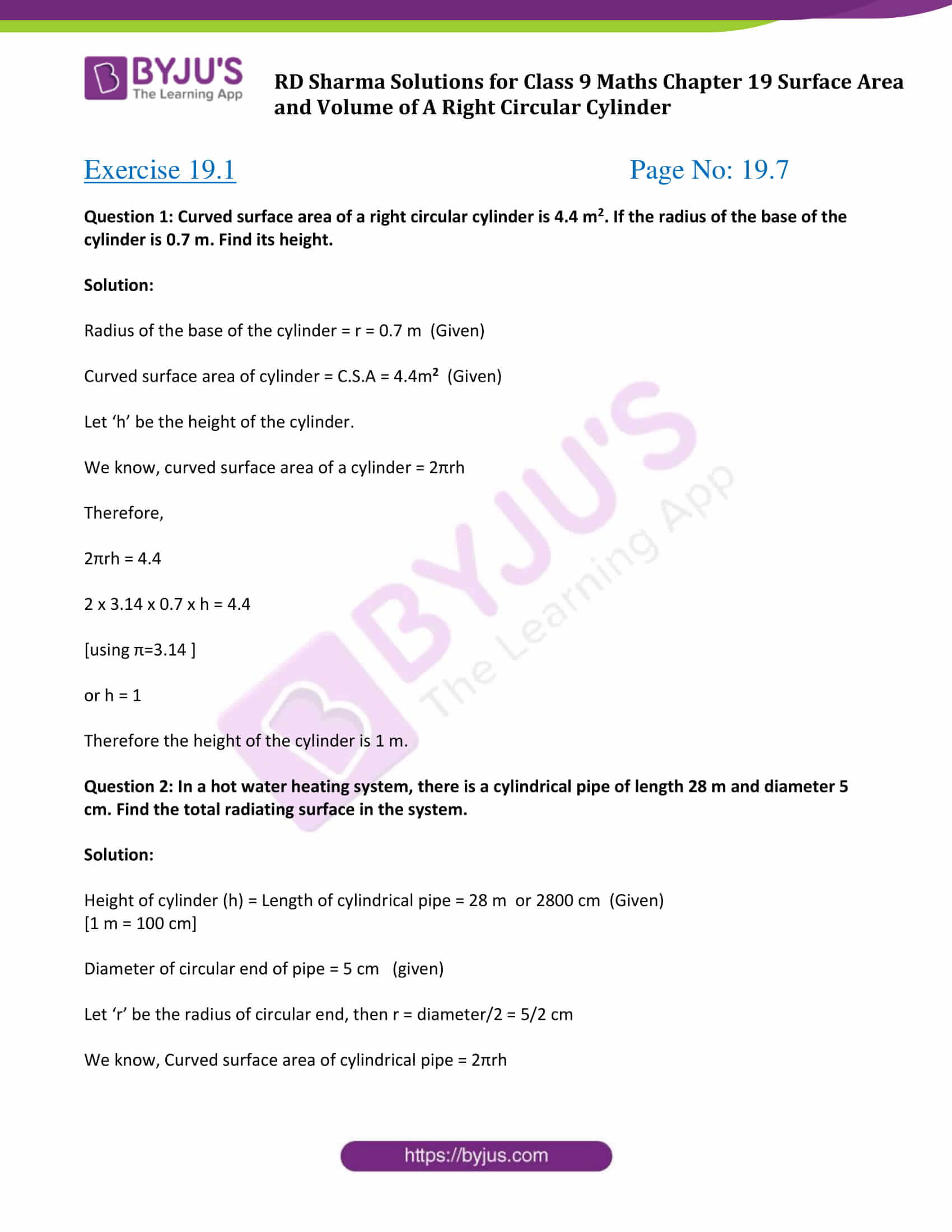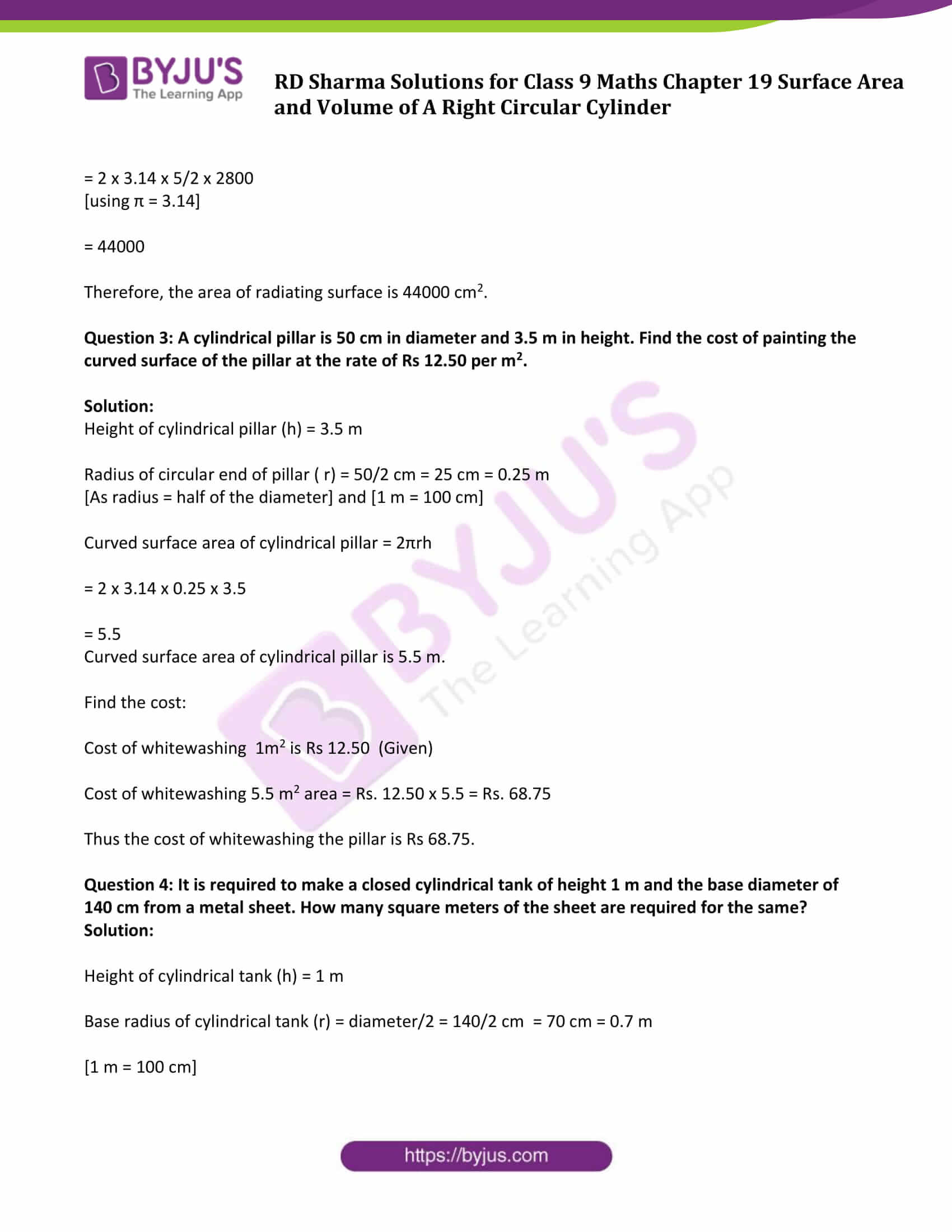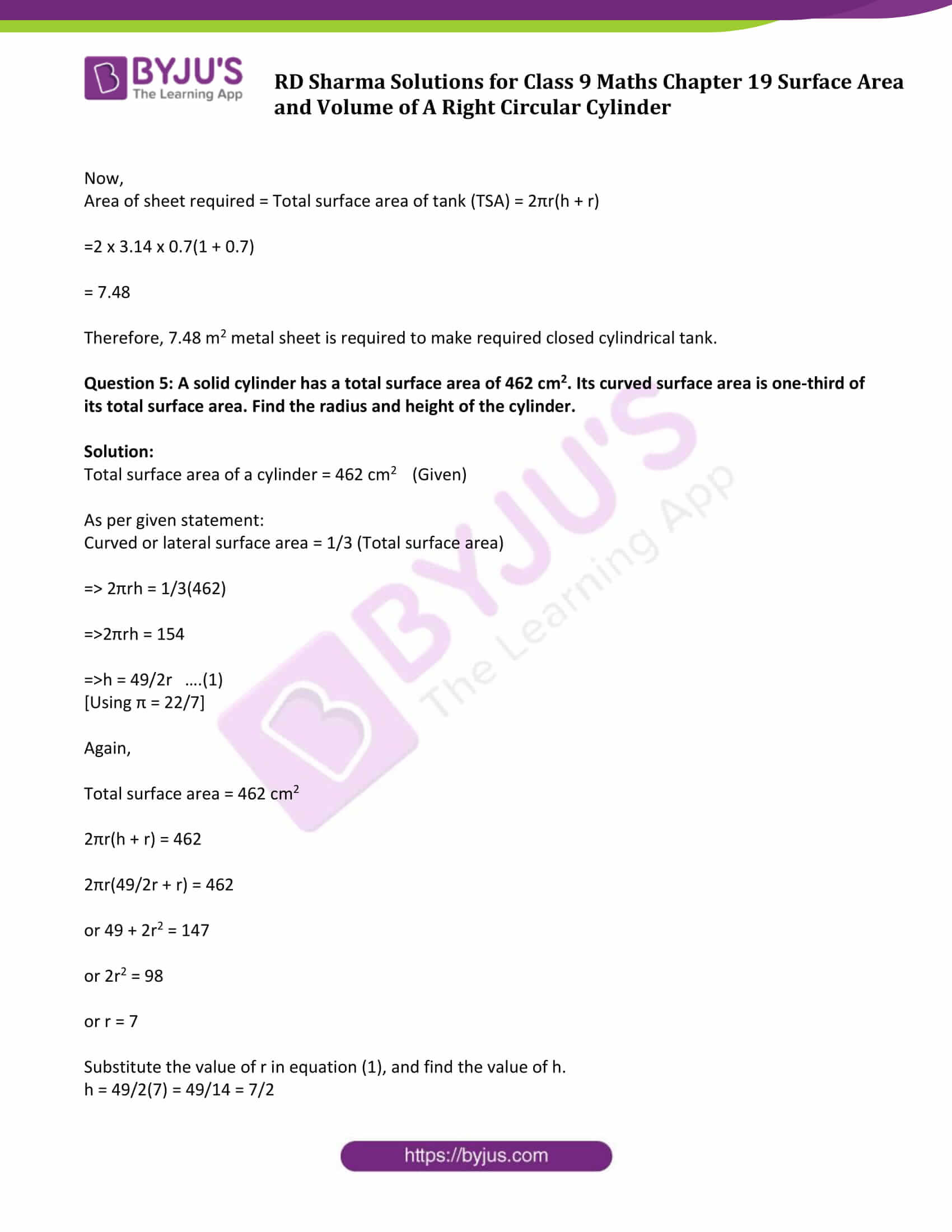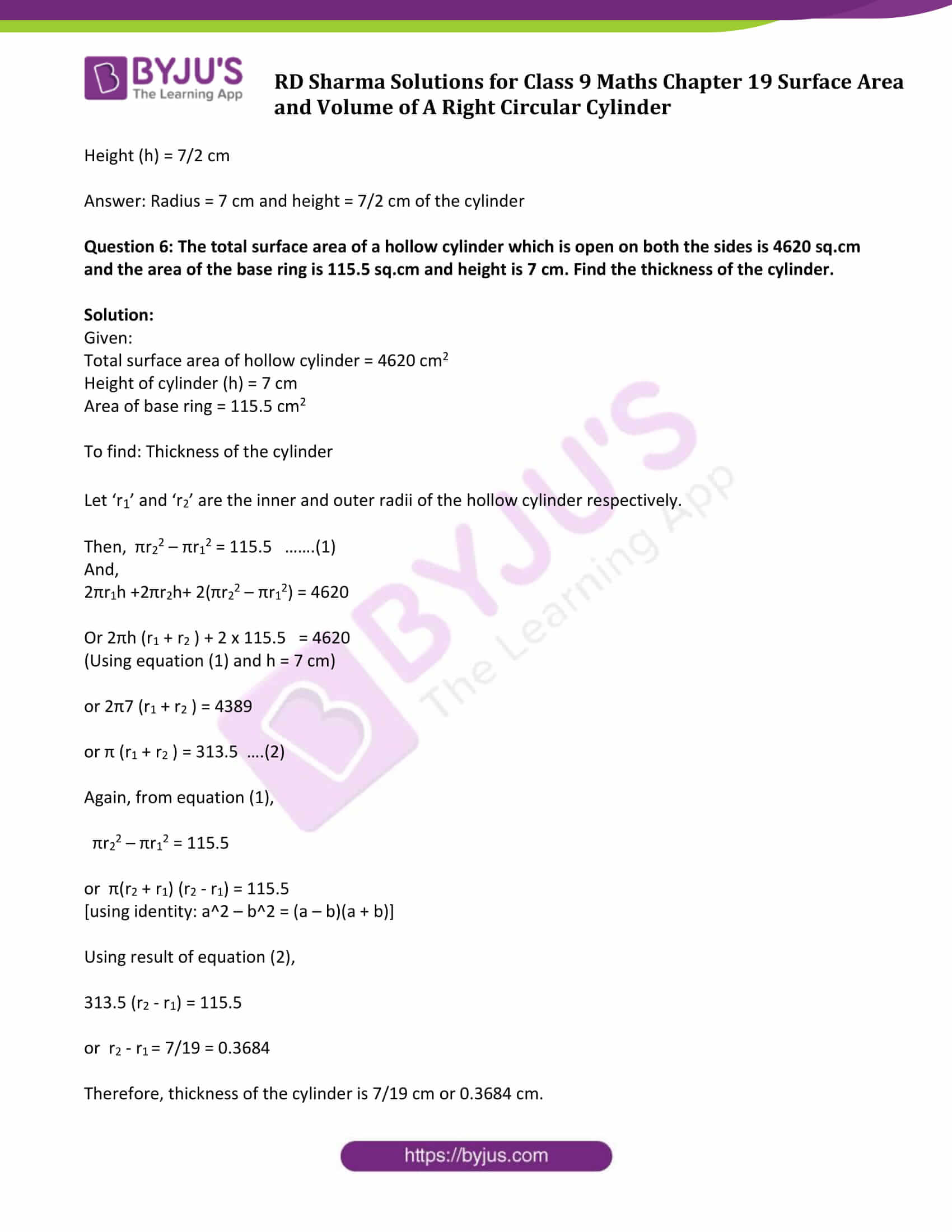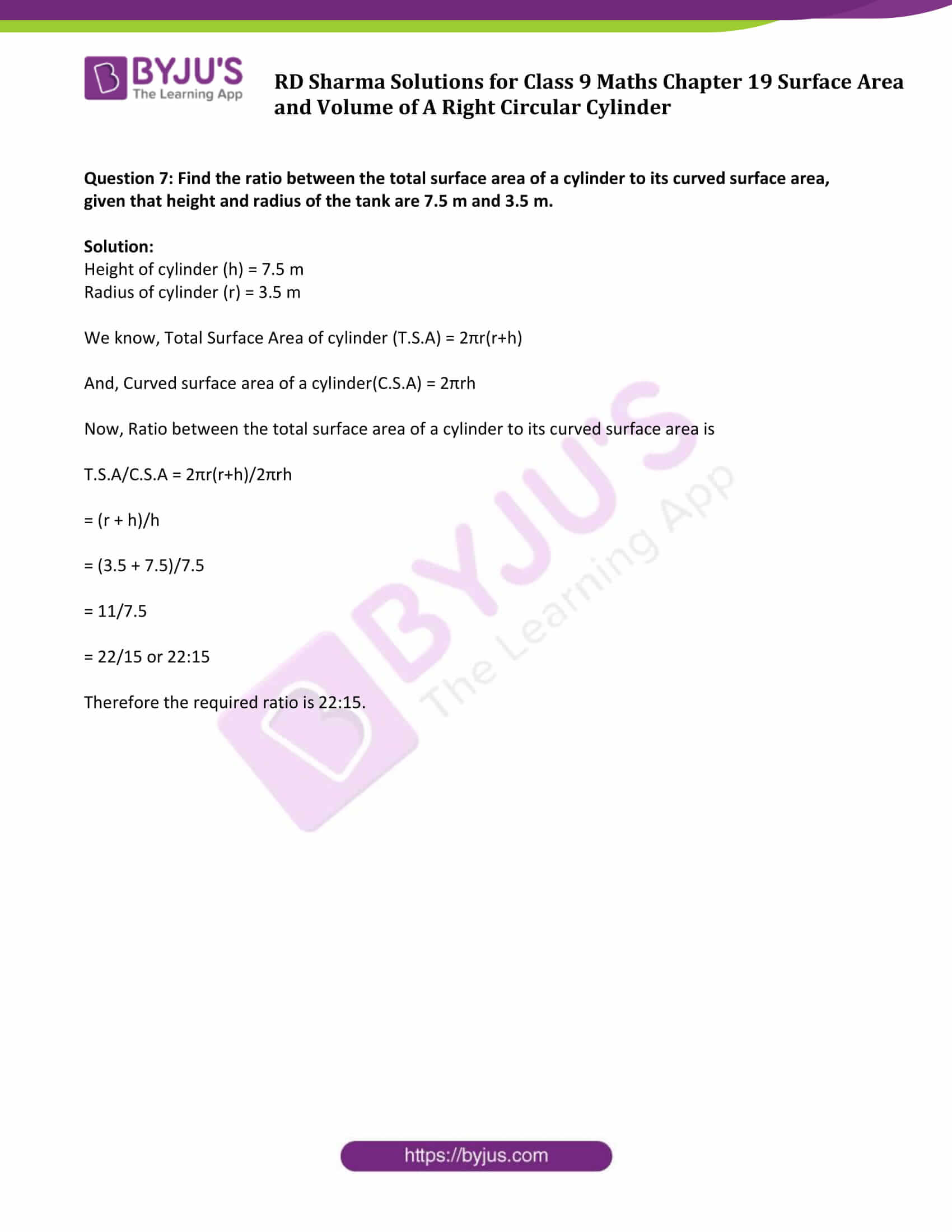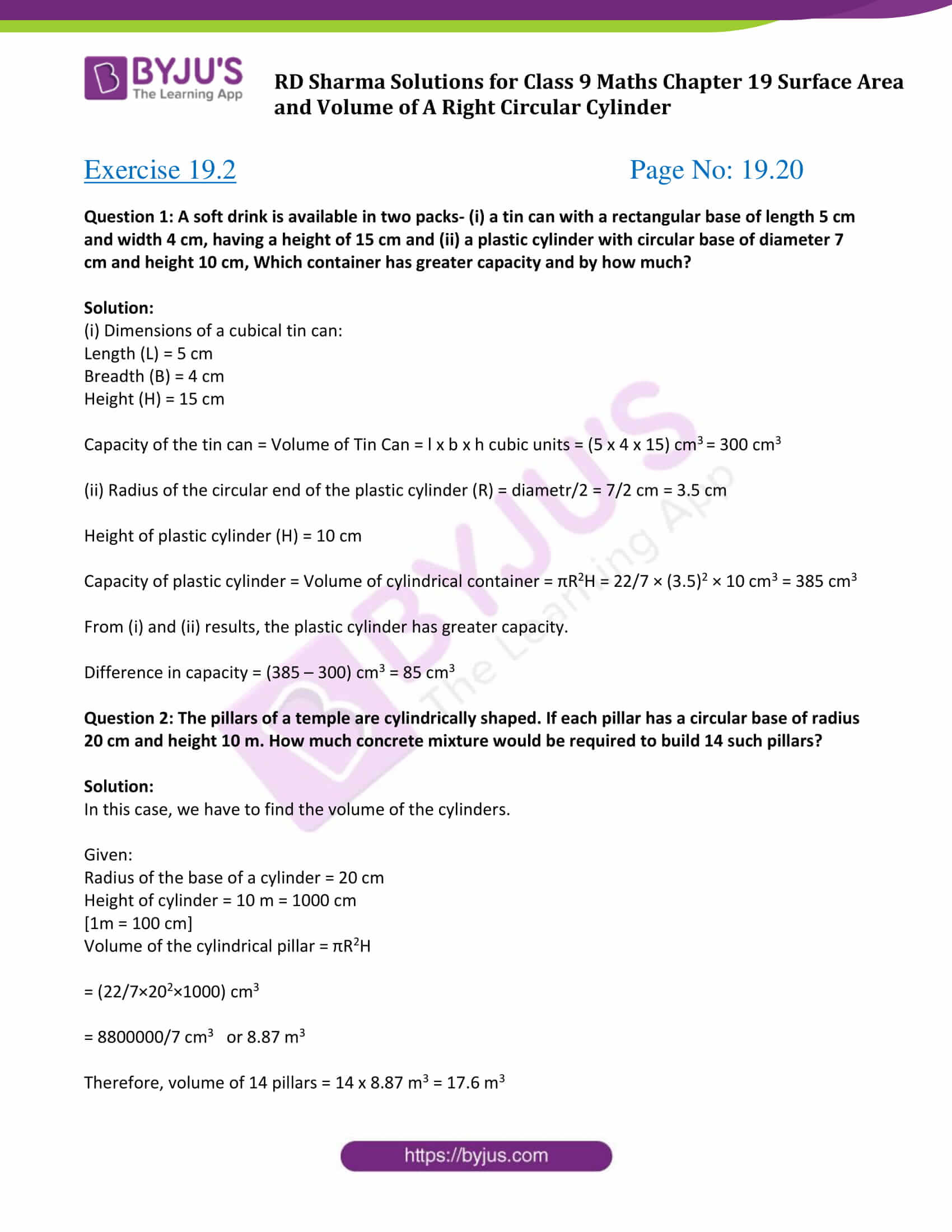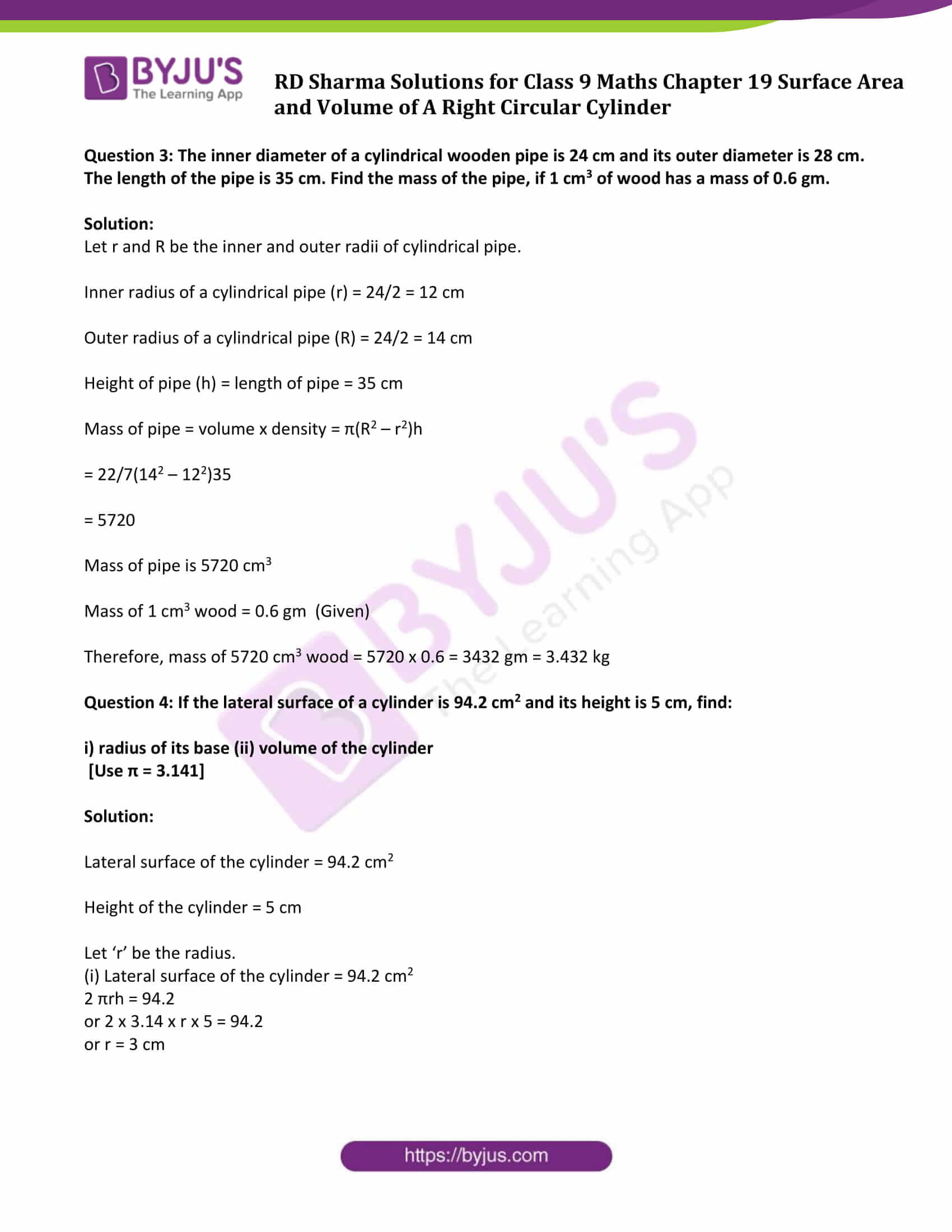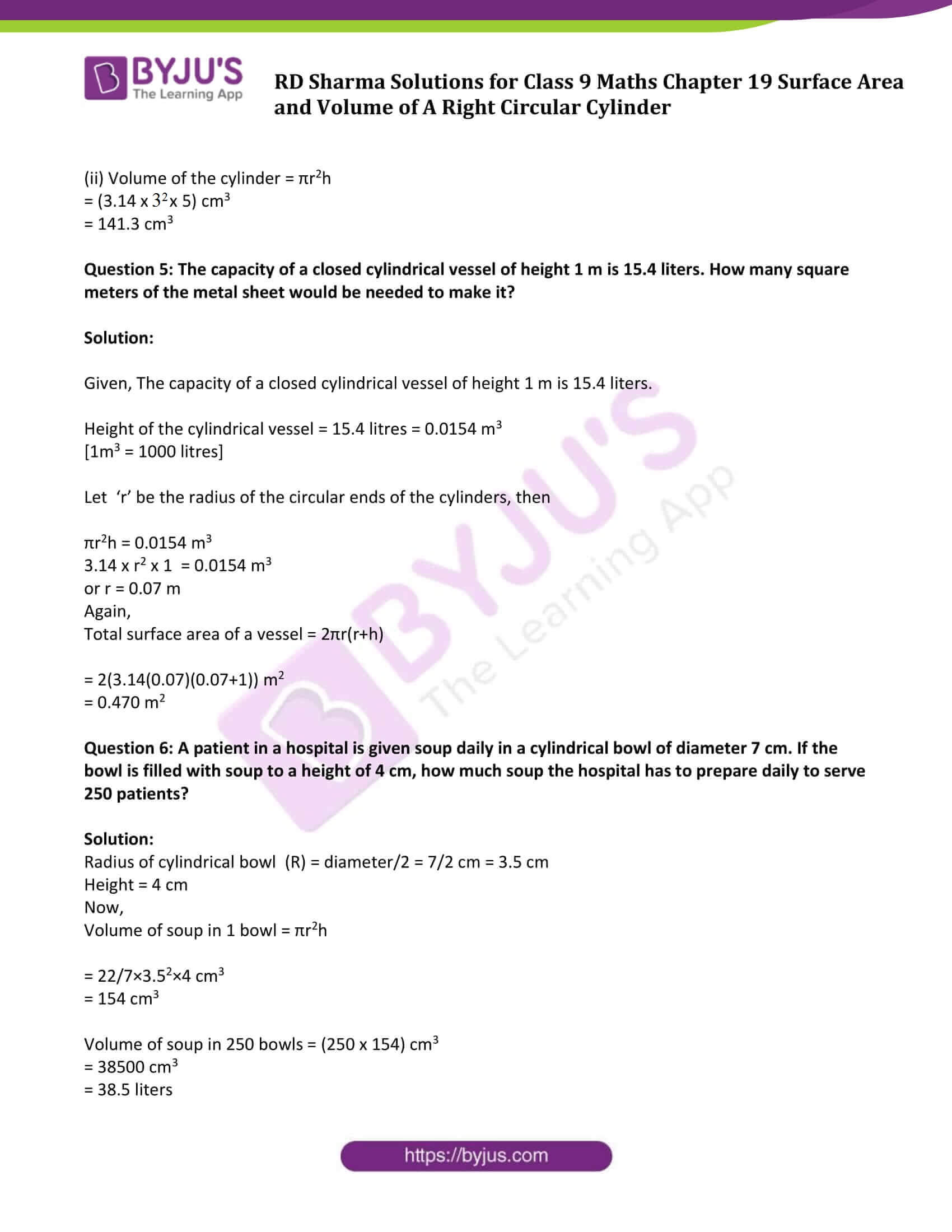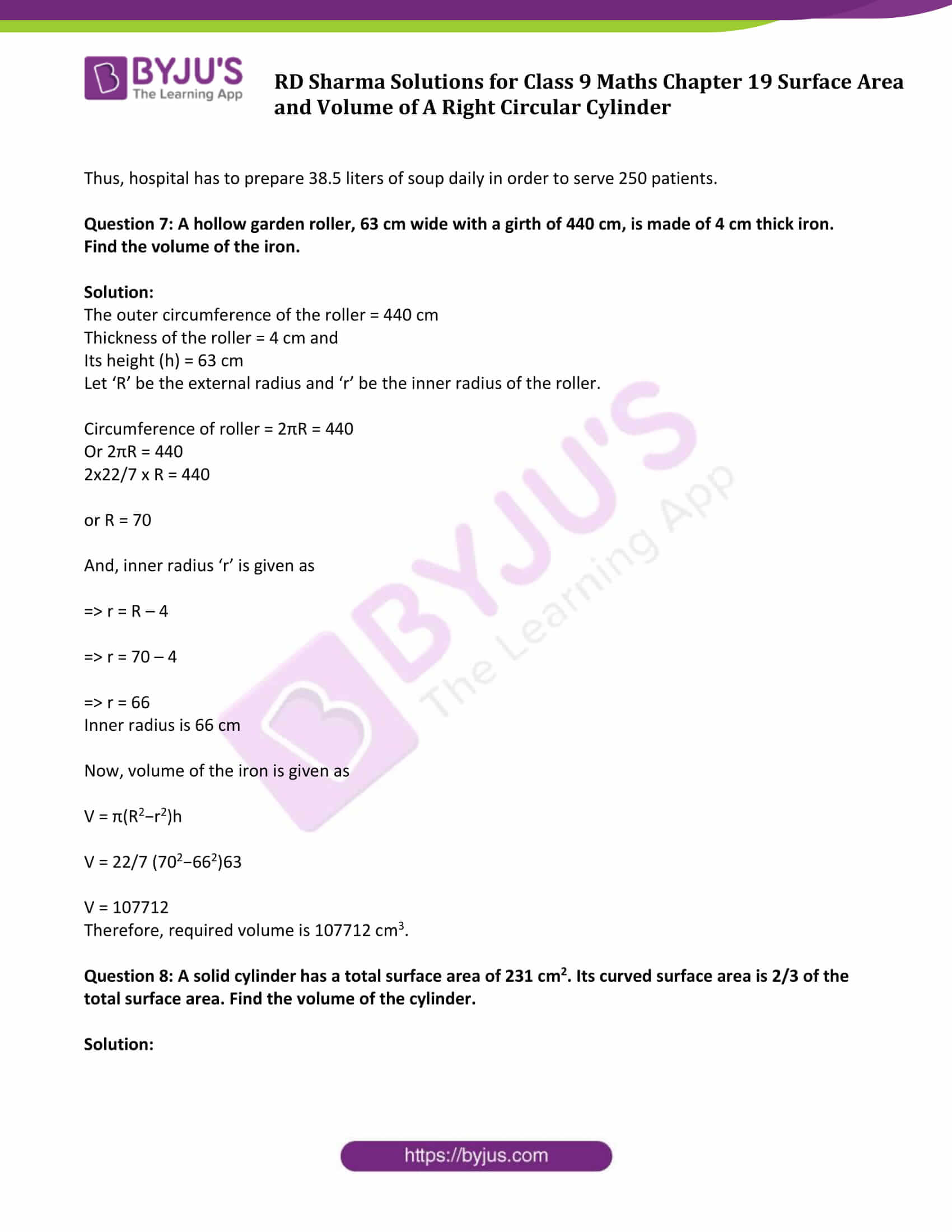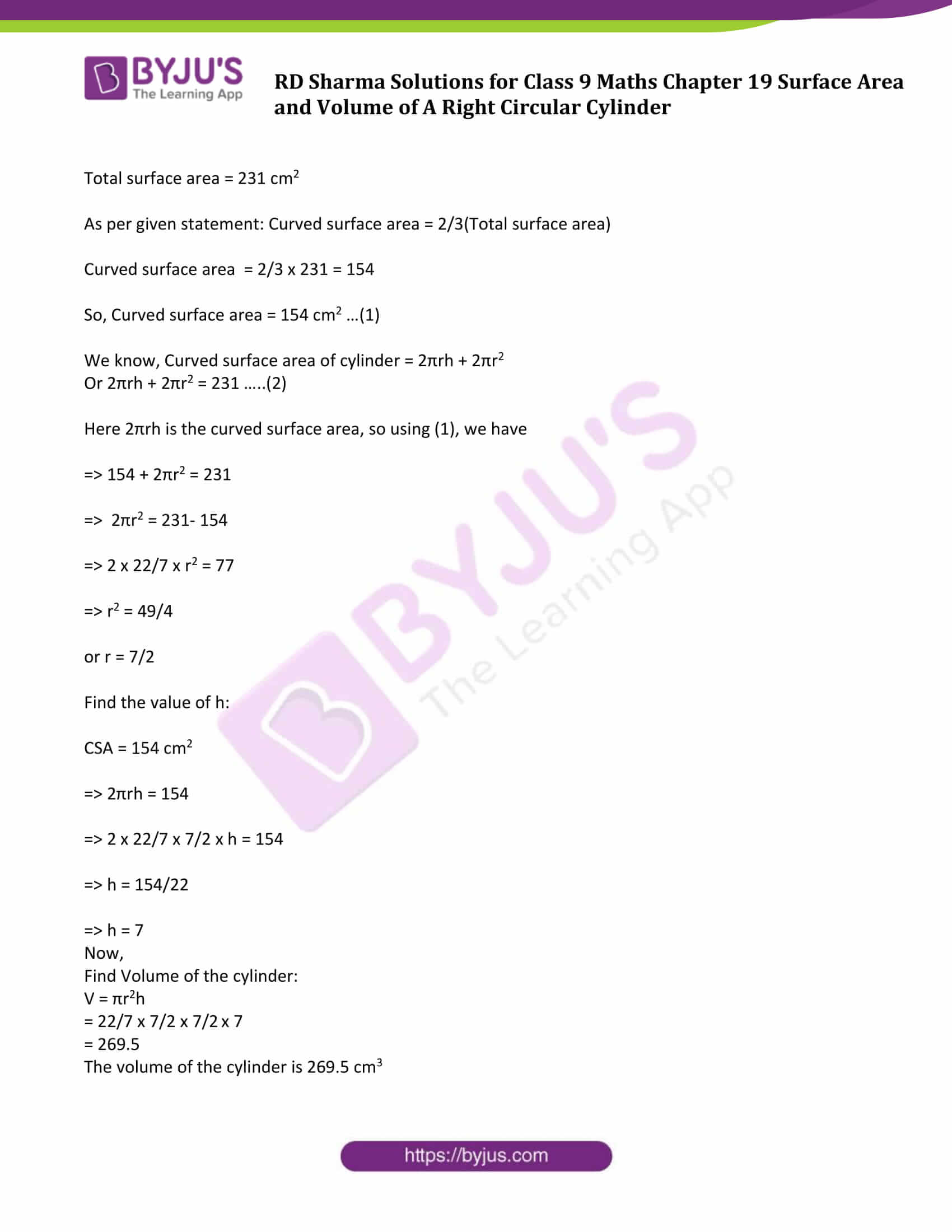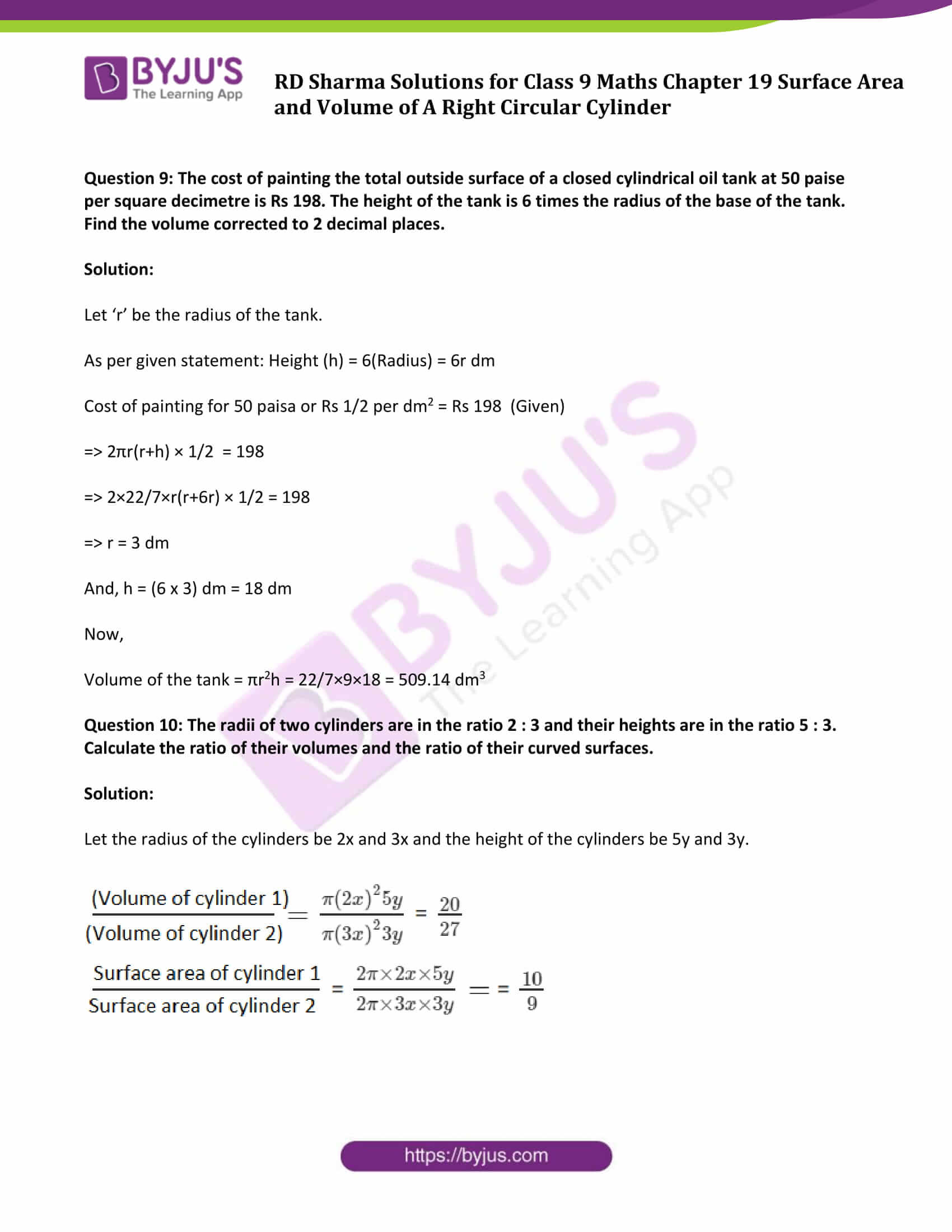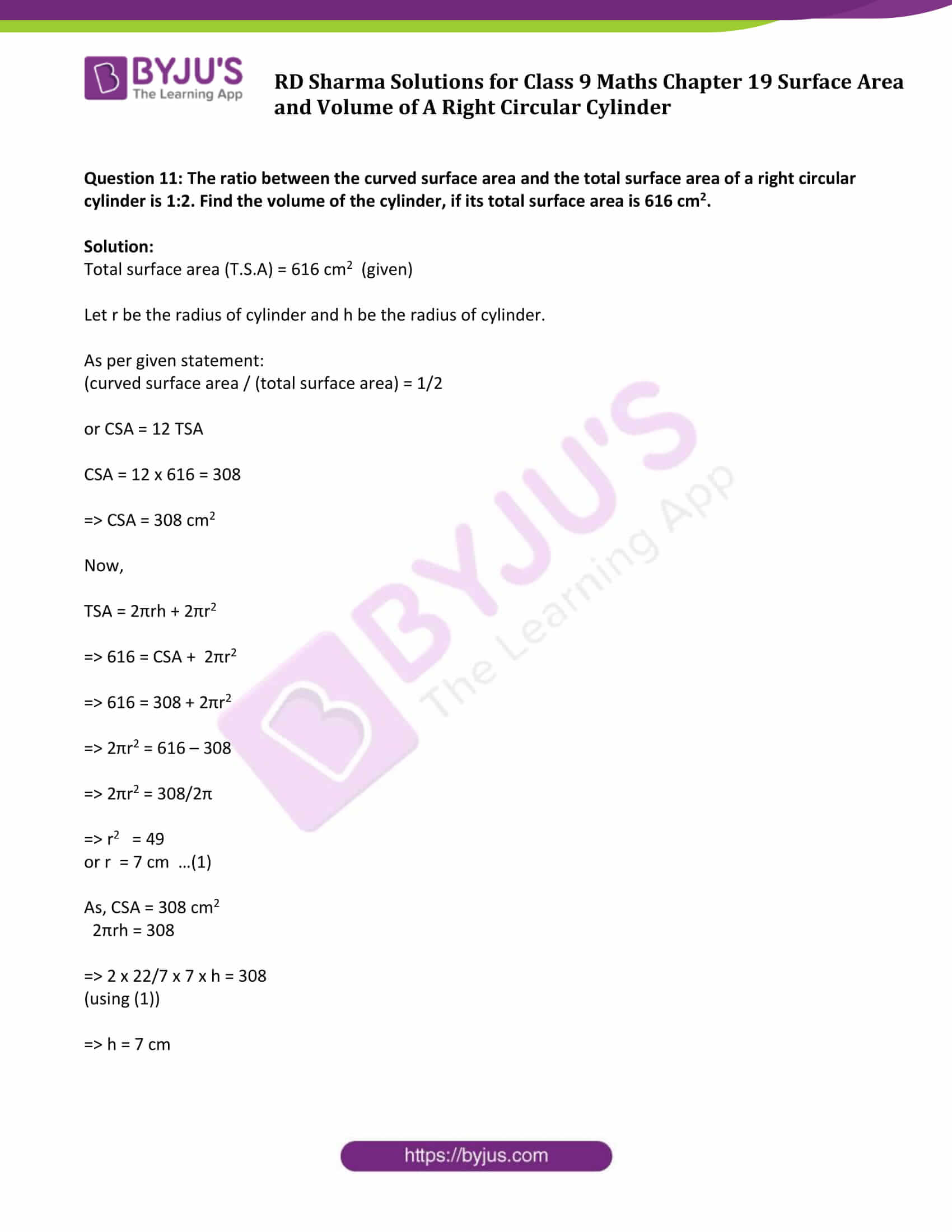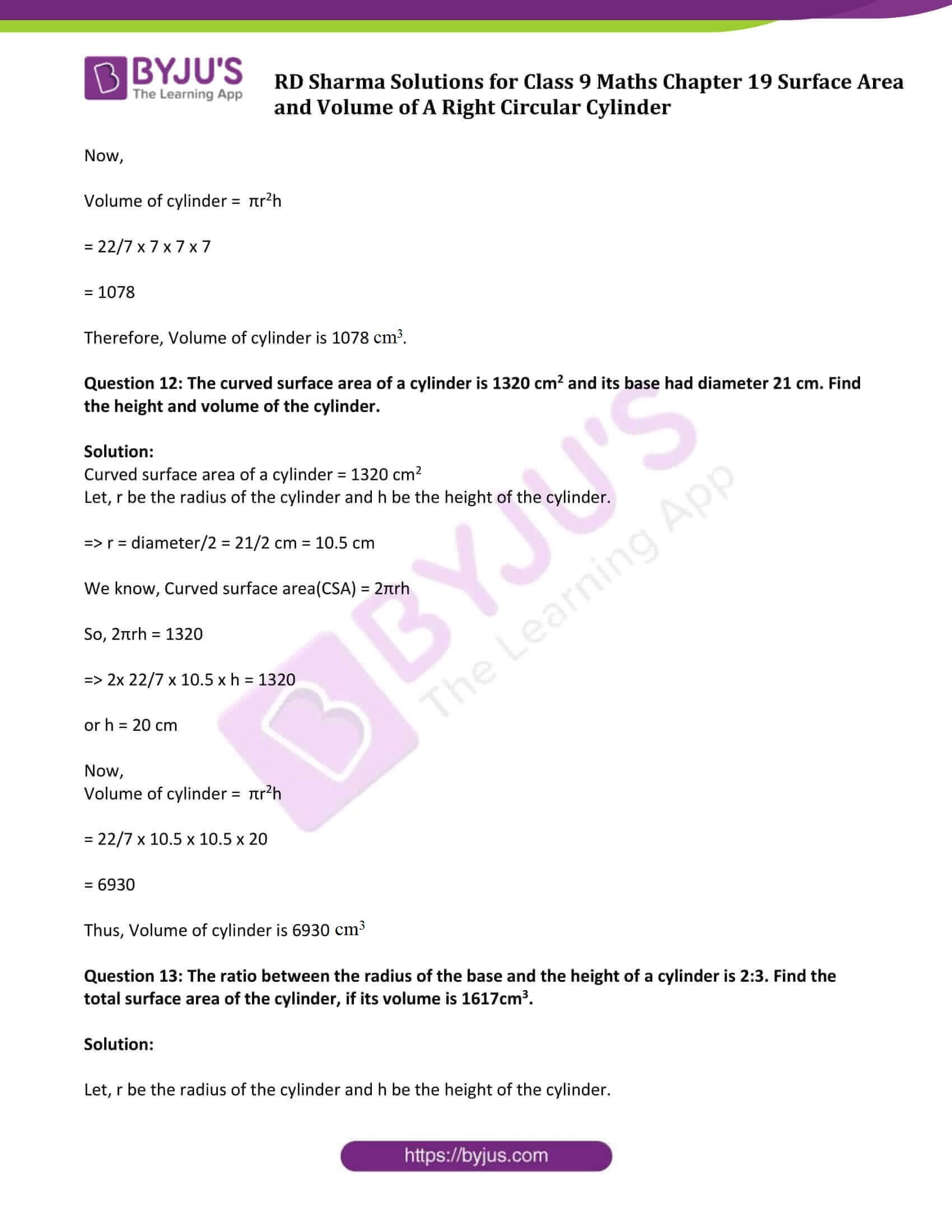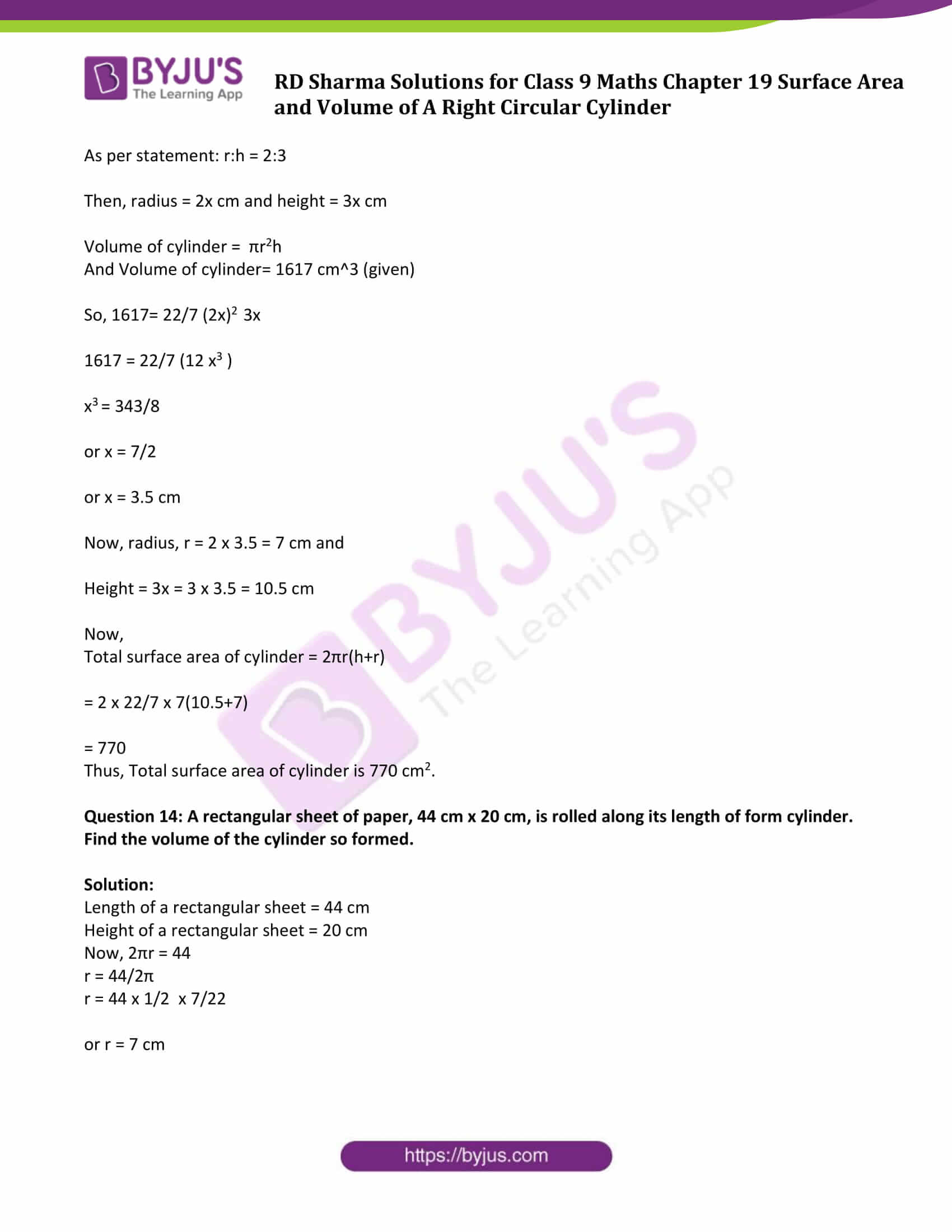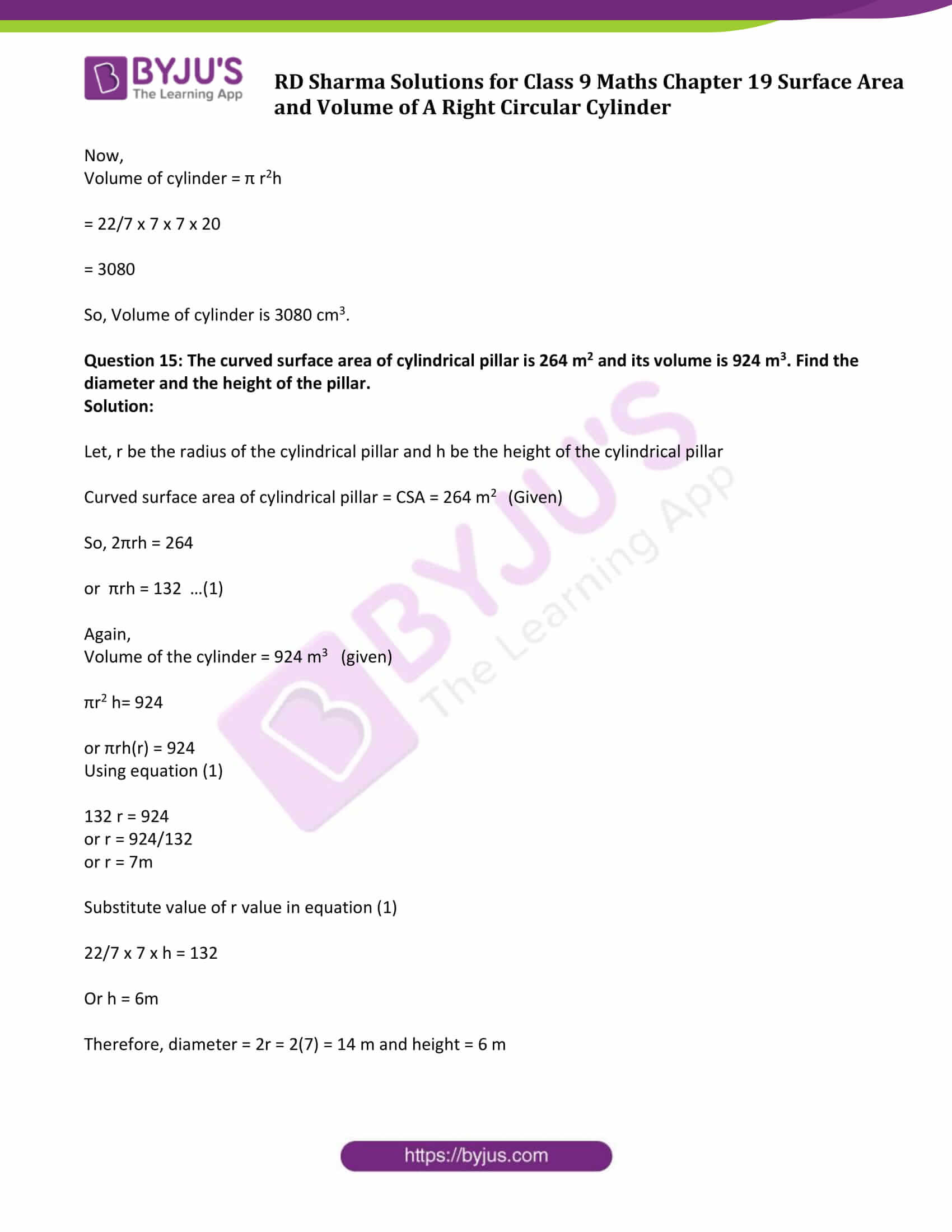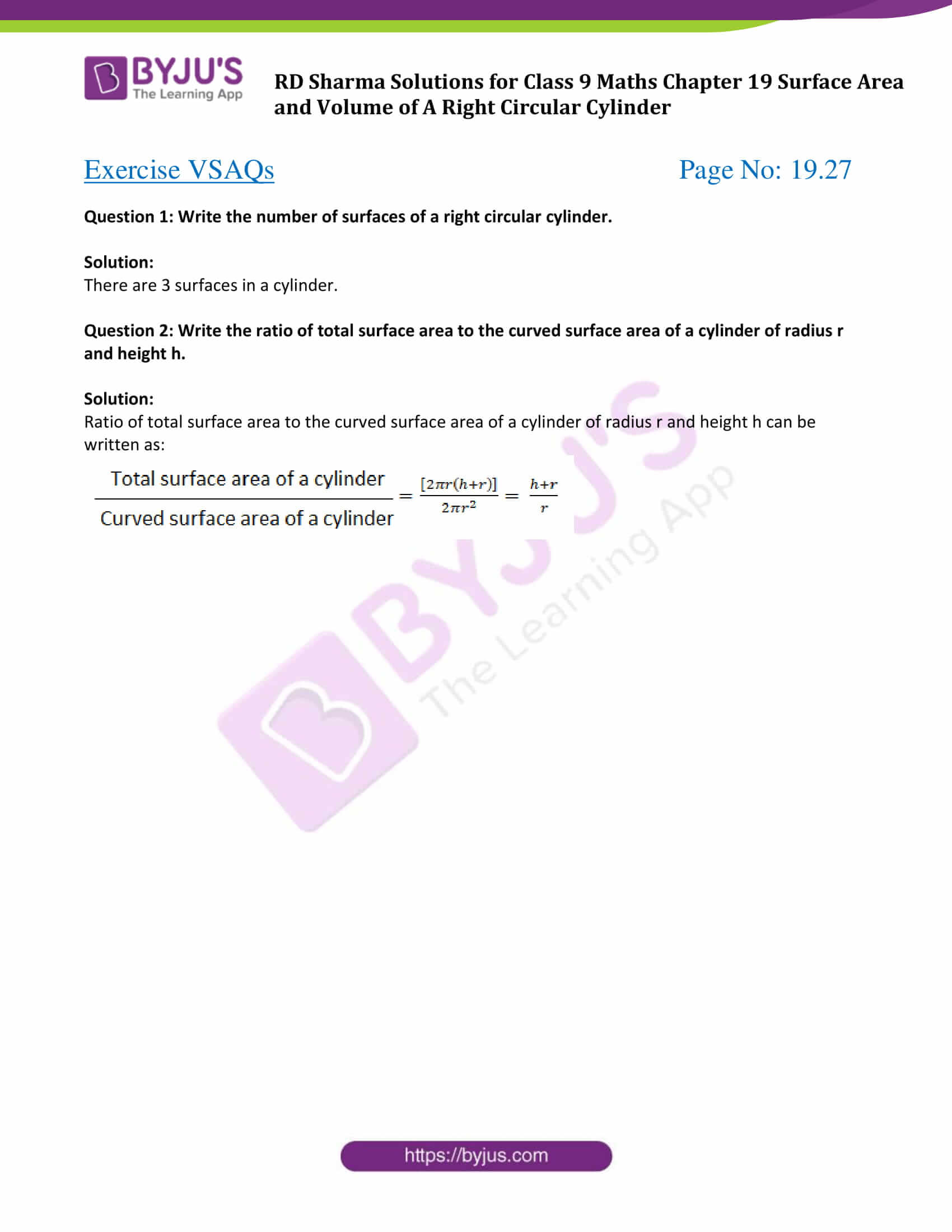### Exercise 19.1 Page No: 19.7

Question 1: Curved surface area of a right circular cylinder is 4.4 m2. If the radius of the base of the cylinder is 0.7 m. Find its height.

Solution:

Radius of the base of the cylinder = r = 0.7 m (Given)

Curved surface area of cylinder = C.S.A = 4.4m2 (Given)

Let ‘h’ be the height of the cylinder.

We know, curved surface area of a cylinder = 2πrh

Therefore,

2πrh = 4.4

2 x 3.14 x 0.7 x h = 4.4

[using π=3.14 ]

or h = 1

Therefore the height of the cylinder is 1 m.

Question 2: In a hot water heating system, there is a cylindrical pipe of length 28 m and diameter 5 cm. Find the total radiating surface in the system.

Solution:

Height of cylinder (h) = Length of cylindrical pipe = 28 m or 2800 cm (Given)

[1 m = 100 cm]

Diameter of circular end of pipe = 5 cm (given)

Let ‘r’ be the radius of circular end, then r = diameter/2 = 5/2 cm

We know, Curved surface area of cylindrical pipe = 2πrh

= 2 x 3.14 x 5/2 x 2800

[using π = 3.14]

= 44000

Therefore, the area of radiating surface is 44000 cm2.

Question 3: A cylindrical pillar is 50 cm in diameter and 3.5 m in height. Find the cost of painting the curved surface of the pillar at the rate of Rs 12.50 per m2.

Solution:

Height of cylindrical pillar (h) = 3.5 m

Radius of circular end of pillar ( r) = 50/2 cm = 25 cm = 0.25 m

[As radius = half of the diameter] and [1 m = 100 cm]

Curved surface area of cylindrical pillar = 2πrh

= 2 x 3.14 x 0.25 x 3.5

= 5.5

Curved surface area of cylindrical pillar is 5.5 m.

Find the cost:

Cost of whitewashing 1m2 is Rs 12.50 (Given)

Cost of whitewashing 5.5 m2 area = Rs. 12.50 x 5.5 = Rs. 68.75

Thus the cost of whitewashing the pillar is Rs 68.75.

Question 4: It is required to make a closed cylindrical tank of height 1 m and the base diameter of 140 cm from a metal sheet. How many square meters of the sheet are required for the same?

Solution:

Height of cylindrical tank (h) = 1 m

Base radius of cylindrical tank (r) = diameter/2 = 140/2 cm = 70 cm = 0.7 m

[1 m = 100 cm]

Now,

Area of sheet required = Total surface area of tank (TSA) = 2πr(h + r)

=2 x 3.14 x 0.7(1 + 0.7)

= 7.48

Therefore, 7.48 m2 metal sheet is required to make required closed cylindrical tank.

Question 5: A solid cylinder has a total surface area of 462 cm2. Its curved surface area is one-third of its total surface area. Find the radius and height of the cylinder.

Solution:

Total surface area of a cylinder = 462 cm2 (Given)

As per given statement:

Curved or lateral surface area = 1/3 (Total surface area)

⇒ 2πrh = 1/3(462)

⇒ 2πrh = 154

⇒ h = 49/2r ….(1)

[Using π = 22/7]

Again,

Total surface area = 462 cm2

2πr(h + r) = 462

2πr(49/2r + r) = 462

or 49 + 2r2 = 147

or 2r2 = 98

or r = 7

Substitute the value of r in equation (1), and find the value of h.

h = 49/2(7) = 49/14 = 7/2

Height (h) = 7/2 cm

Answer: Radius = 7 cm and height = 7/2 cm of the cylinder

Question 6: The total surface area of a hollow cylinder which is open on both the sides is 4620 sq.cm and the area of the base ring is 115.5 sq.cm and height is 7 cm. Find the thickness of the cylinder.

Solution:

Given:

Total surface area of hollow cylinder = 4620 cm2

Height of cylinder (h) = 7 cm

Area of base ring = 115.5 cm2

To find: Thickness of the cylinder

Let ‘r1’ and ‘r2’ are the inner and outer radii of the hollow cylinder respectively.

Then, πr22 – πr12 = 115.5 …….(1)

And,

2πr1h +2πr2h+ 2(πr22 – πr12) = 4620

Or 2πh (r1 + r2 ) + 2 x 115.5 = 4620

(Using equation (1) and h = 7 cm)

or 2π7 (r1 + r2 ) = 4389

or π (r1 + r2 ) = 313.5 ….(2)

Again, from equation (1),

πr22 – πr12 = 115.5

or π(r2 + r1) (r2 – r1) = 115.5

[using identity: a^2 – b^2 = (a – b)(a + b)]

Using result of equation (2),

313.5 (r2 – r1) = 115.5

or r2 – r1 = 7/19 = 0.3684

Therefore, thickness of the cylinder is 7/19 cm or 0.3684 cm.

Question 7: Find the ratio between the total surface area of a cylinder to its curved surface area, given that height and radius of the tank are 7.5 m and 3.5 m.

Solution:

Height of cylinder (h) = 7.5 m

Radius of cylinder (r) = 3.5 m

We know, Total Surface Area of cylinder (T.S.A) = 2πr(r+h)

And, Curved surface area of a cylinder(C.S.A) = 2πrh

Now, Ratio between the total surface area of a cylinder to its curved surface area is

T.S.A/C.S.A = 2πr(r+h)/2πrh

= (r + h)/h

= (3.5 + 7.5)/7.5

= 11/7.5

= 22/15 or 22:15

Therefore the required ratio is 22:15.

### Exercise 19.2 Page No: 19.20

Question 1: A soft drink is available in two packs- (i) a tin can with a rectangular base of length 5 cm and width 4 cm, having a height of 15 cm and (ii) a plastic cylinder with circular base of diameter 7 cm and height 10 cm, Which container has greater capacity and by how much?

Solution:

(i) Dimensions of a cubical tin can:

Length (L) = 5 cm

Height (H) = 15 cm

Capacity of the tin can = Volume of Tin Can = l x b x h cubic units = (5 x 4 x 15) cm3 = 300 cm3

(ii) Radius of the circular end of the plastic cylinder (R) = diametr/2 = 7/2 cm = 3.5 cm

Height of plastic cylinder (H) = 10 cm

Capacity of plastic cylinder = Volume of cylindrical container = πR2H = 22/7 × (3.5)2 × 10 cm3 = 385 cm3

From (i) and (ii) results, the plastic cylinder has greater capacity.

Difference in capacity = (385 – 300) cm3 = 85 cm3

Question 2: The pillars of a temple are cylindrically shaped. If each pillar has a circular base of radius 20 cm and height 10 m. How much concrete mixture would be required to build 14 such pillars?

Solution:

In this case, we have to find the volume of the cylinders.

Given:

Radius of the base of a cylinder = 20 cm

Height of cylinder = 10 m = 1000 cm

[1m = 100 cm]

Volume of the cylindrical pillar = πR2H

= (22/7×202×1000) cm3

= 8800000/7 cm3 or 8.87 m3

Therefore, volume of 14 pillars = 14 x 8.87 m3 = 17.6 m3

Question 3: The inner diameter of a cylindrical wooden pipe is 24 cm and its outer diameter is 28 cm. The length of the pipe is 35 cm. Find the mass of the pipe, if 1 cm3 of wood has a mass of 0.6 gm.

Solution:

Let r and R be the inner and outer radii of cylindrical pipe.

Inner radius of a cylindrical pipe (r) = 24/2 = 12 cm

Outer radius of a cylindrical pipe (R) = 24/2 = 14 cm

Height of pipe (h) = length of pipe = 35 cm

Mass of pipe = volume x density = π(R2 – r2)h

= 22/7(142 – 122)35

= 5720

Mass of pipe is 5720 cm3

Mass of 1 cm3 wood = 0.6 gm (Given)

Therefore, mass of 5720 cm3 wood = 5720 x 0.6 = 3432 gm = 3.432 kg

Question 4: If the lateral surface of a cylinder is 94.2 cm2 and its height is 5 cm, find:

i) radius of its base (ii) volume of the cylinder

[Use π = 3.141]

Solution:

Lateral surface of the cylinder = 94.2 cm2

Height of the cylinder = 5 cm

(i) Lateral surface of the cylinder = 94.2 cm2

2 πrh = 94.2

or 2 x 3.14 x r x 5 = 94.2

or r = 3 cm

(ii) Volume of the cylinder = πr2h

= (3.14 x 32 x 5) cm3

= 141.3 cm3

Question 5: The capacity of a closed cylindrical vessel of height 1 m is 15.4 liters. How many square meters of the metal sheet would be needed to make it?

Solution:

Given, The capacity of a closed cylindrical vessel of height 1 m is 15.4 liters.

Height of the cylindrical vessel = 15.4 litres = 0.0154 m3

[1m3 = 1000 litres]

Let ‘r’ be the radius of the circular ends of the cylinders, then

πr2h = 0.0154 m3

3.14 x r2 x 1 = 0.0154 m3

or r = 0.07 m

Again,

Total surface area of a vessel = 2πr(r+h)

= 2(3.14(0.07)(0.07+1)) m2

= 0.470 m2

Question 6: A patient in a hospital is given soup daily in a cylindrical bowl of diameter 7 cm. If the bowl is filled with soup to a height of 4 cm, how much soup the hospital has to prepare daily to serve 250 patients?

Solution:

Radius of cylindrical bowl (R) = diameter/2 = 7/2 cm = 3.5 cm

Height = 4 cm

Now,

Volume of soup in 1 bowl = πr2h

= 22/7×3.52×4 cm3

= 154 cm3

Volume of soup in 250 bowls = (250 x 154) cm3

= 38500 cm3

= 38.5 liters

Thus, hospital has to prepare 38.5 liters of soup daily in order to serve 250 patients.

Question 7: A hollow garden roller, 63 cm wide with a girth of 440 cm, is made of 4 cm thick iron. Find the volume of the iron.

Solution:

The outer circumference of the roller = 440 cm

Thickness of the roller = 4 cm and

Its height (h) = 63 cm

Let ‘R’ be the external radius and ‘r’ be the inner radius of the roller.

Circumference of roller = 2πR = 440

Or 2πR = 440

2×22/7 x R = 440

or R = 70

And, inner radius ‘r’ is given as

⇒ r = R – 4

⇒ r = 70 – 4

⇒ r = 66

Now, volume of the iron is given as

V = π(R2−r2)h

V = 22/7 (702−662)63

V = 107712

Therefore, required volume is 107712 cm3.

Question 8: A solid cylinder has a total surface area of 231 cm2. Its curved surface area is 2/3 of the total surface area. Find the volume of the cylinder.

Solution:

Total surface area = 231 cm2

As per given statement: Curved surface area = 2/3(Total surface area)

Curved surface area = 2/3 x 231 = 154

So, Curved surface area = 154 cm2 …(1)

We know, Curved surface area of cylinder = 2πrh + 2πr2

Or 2πrh + 2πr2 = 231 …..(2)

Here 2πrh is the curved surface area, so using (1), we have

⇒ 154 + 2πr2 = 231

⇒ 2πr2 = 231- 154

⇒ 2 x 22/7 x r2 = 77

⇒ r2 = 49/4

or r = 7/2

Find the value of h:

CSA = 154 cm2

⇒ 2πrh = 154

⇒ 2 x 22/7 x 7/2 x h = 154

⇒ h = 154/22

⇒ h = 7

Now,

Find Volume of the cylinder:

V = πr2h

= 22/7 x 7/2 x 7/2 x 7

= 269.5

The volume of the cylinder is 269.5 cm3

Question 9: The cost of painting the total outside surface of a closed cylindrical oil tank at 50 paise per square decimetre is Rs 198. The height of the tank is 6 times the radius of the base of the tank. Find the volume corrected to 2 decimal places.

Solution:

Let ‘r’ be the radius of the tank.

As per given statement: Height (h) = 6(Radius) = 6r dm

Cost of painting for 50 paisa or Rs 1/2 per dm2 = Rs 198 (Given)

⇒ 2πr(r+h) × 1/2 = 198

⇒ 2×22/7×r(r+6r) × 1/2 = 198

⇒ r = 3 dm

And, h = (6 x 3) dm = 18 dm

Now,

Volume of the tank = πr2h = 22/7×9×18 = 509.14 dm3

Question 10: The radii of two cylinders are in the ratio 2 : 3 and their heights are in the ratio 5 : 3. Calculate the ratio of their volumes and the ratio of their curved surfaces.

Solution:

Let the radius of the cylinders be 2x and 3x and the height of the cylinders be 5y and 3y.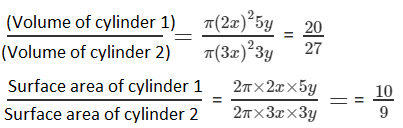Question 11: The ratio between the curved surface area and the total surface area of a right circular cylinder is 1:2. Find the volume of the cylinder, if its total surface area is 616 cm2.

Solution:

Total surface area (T.S.A) = 616 cm2 (given)

Let r be the radius of cylinder and h be the radius of cylinder.

As per given statement:

(curved surface area / (total surface area) = 1/2

or CSA = 12 TSA

CSA = 12 x 616 = 308

⇒ CSA = 308 cm2

Now,

TSA = 2πrh + 2πr2

⇒ 616 = CSA + 2πr2

⇒ 616 = 308 + 2πr2

⇒ 2πr2 = 616 – 308

⇒ 2πr2 = 308/2π

⇒ r2 = 49

or r = 7 cm …(1)

As, CSA = 308 cm2

2πrh = 308

⇒ 2 x 22/7 x 7 x h = 308

(using (1))

⇒ h = 7 cm

Now,

Volume of cylinder = πr2h

= 22/7 x 7 x 7 x 7

= 1078

Therefore, Volume of cylinder is 1078 cm3.

Question 12: The curved surface area of a cylinder is 1320 cm2 and its base had diameter 21 cm. Find the height and volume of the cylinder.

Solution:

Curved surface area of a cylinder = 1320 cm2

Let, r be the radius of the cylinder and h be the height of the cylinder.

⇒ r = diameter/2 = 21/2 cm = 10.5 cm

We know, Curved surface area(CSA) = 2πrh

So, 2πrh = 1320

⇒ 2x 22/7 x 10.5 x h = 1320

or h = 20 cm

Now,

Volume of cylinder = πr2h

= 22/7 x 10.5 x 10.5 x 20

= 6930

Thus, Volume of cylinder is 6930 cm3.

Question 13: The ratio between the radius of the base and the height of a cylinder is 2:3. Find the total surface area of the cylinder, if its volume is 1617cm3.

Solution:

Let, r be the radius of the cylinder and h be the height of the cylinder.

As per statement: r:h = 2:3

Then, radius = 2x cm and height = 3x cm

Volume of cylinder = πr2h

And Volume of cylinder= 1617 cm^3 (given)

So, 1617= 22/7 (2x)2 3x

1617 = 22/7 (12 x3 )

x3 = 343/8

or x = 7/2

or x = 3.5 cm

Now, radius, r = 2 x 3.5 = 7 cm and

Height = 3x = 3 x 3.5 = 10.5 cm

Now,

Total surface area of cylinder = 2πr(h+r)

= 2 x 22/7 x 7(10.5+7)

= 770

Thus, Total surface area of cylinder is 770 cm2.

Question 14: A rectangular sheet of paper, 44 cm x 20 cm, is rolled along its length of form cylinder. Find the volume of the cylinder so formed.

Solution:

Length of a rectangular sheet = 44 cm

Height of a rectangular sheet = 20 cm

Now, 2πr = 44

r = 44/2π

r = 44 x 1/2 x 7/22

or r = 7 cm

Now,

Volume of cylinder = π r2h

= 22/7 x 7 x 7 x 20

= 3080

So, Volume of cylinder is 3080 cm3.

Question 15: The curved surface area of cylindrical pillar is 264 m2 and its volume is 924 m3. Find the diameter and the height of the pillar.

Solution:

Let, r be the radius of the cylindrical pillar and h be the height of the cylindrical pillar

Curved surface area of cylindrical pillar = CSA = 264 m2 (Given)

So, 2πrh = 264

or πrh = 132 …(1)

Again,

Volume of the cylinder = 924 m3 (given)

πr2 h= 924

or πrh(r) = 924

Using equation (1)

132 r = 924

or r = 924/132

or r = 7m

Substitute value of r value in equation (1)

22/7 x 7 x h = 132

Or h = 6m

Therefore, diameter = 2r = 2(7) = 14 m and height = 6 m

### Exercise VSAQs Page No: 19.27

Question 1: Write the number of surfaces of a right circular cylinder.

Solution:

There are 3 surfaces in a cylinder.

Question 2: Write the ratio of total surface area to the curved surface area of a cylinder of radius r and height h.

Solution:

Ratio of total surface area to the curved surface area of a cylinder of radius r and height h can be written as: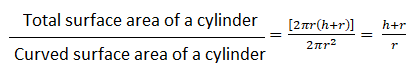### RD Sharma Solutions for Class 9 Maths Chapter 19 Surface Area and Volume of A Right Circular Cylinder

In this 19th Chapter of Class 9 RD Sharma Solutions students will study important concepts listed below:

• Right Circular Cylinder Introduction
• Some important terms definition – Base, Axis, Radius, Height and Lateral Surface.
• Surface Area of a Cylinder
• Volume of a Cylinder

Here is the formula which is applied to calculate the surface area and volume of a circular cylinder.

• Surface area of a cylinder = 2πr (r + h) and
• Volume of a cylinder = πr2h Where r = radius of the cylinder and h = height of the cylinder.

## Frequently Asked Questions on RD Sharma Solutions for Class 9 Maths Chapter 19

### What is the main aim of RD Sharma Solutions for Class 9 Maths Chapter 19?

The students are encouraged to go through RD Sharma Solutions for Class 9 Maths Chapter 19 thoroughly before the final exams to score well and intensify their problem-solving abilities. Understanding the concepts and clearing the doubts immediately during self-study is the main aim of these solutions.

### What are the key benefits of learning RD Sharma Solutions for Class 9 Maths Chapter 19?

Key benefits of RD Sharma Solutions for Class 9 Maths Chapter 19 are provided here:
1. Students can easily access the solutions for each exercise from the chapters available.
2. The solutions also provide graphs and illustrations that help to understand the concepts clearly.
3. These solutions are prepared by BYJU’S expert team focusing on accuracy.

### Why should we refer to RD Sharma Solutions for Class 9 Maths Chapter 19?

Students must refer to RD Sharma Solutions for Class 9 Maths Chapter 19 in gaining knowledge and for better guidance to solve the problems effectively. Regular practice of textbook problems in this chapter will assure that students can score good marks in the exams.